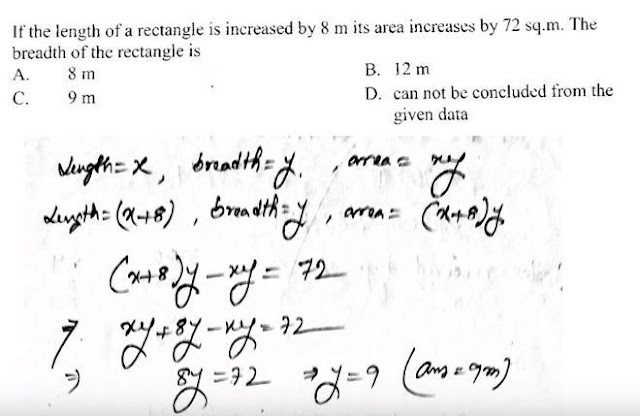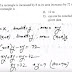Maths coaching for govt jobs competitive exams - Welcome students to www.Coaching123.in ! As we all know that Maths is a crucial subject which comes in all govt jobs competitive exam, whether it be SSC (staff selection commission), IBPS banking, RRB railways, IAS, or any other state/central govt jobs.

Here at Coaching123.in  we are are starting a special Maths class program where we will discuss all types of Maths questions and answers previously asked in govt jobs competitive exams. This will help you to clear your doubts in Maths subject.

## Maths coaching for govt jobs competitive exams - SET 1

Question 1 : If the length of a rectangle is increased by 8 m, and its area increased by 72 sq.m. Then the breadth of the rectangle is ?

a. 8 m
b. 12 m
c. 9 m
d. can not be concluded from the given data

Ans. c

Explanation -Question 2 : Ratio of Principal and yearly amount is 16:17. Then the rate of interest per annum is ?

a. 12 1/2 %
b. 6 1/4 %
c. 5 15/17 %
d. 10 %

Ans. b

Explanation -Question 3 : Area of rectangle is 24 sq. cm. and perimeter is 20 cm. Then the length of diagonal is?

a. 2 7
b. 2 11
c. 2 17
d. 2 13

Ans. d

Question 4 : Ratio of Cost price and Selling price is 5:4. Then what will be the loss percentage ?

a. 15%
b. 20%
c. 9%
d. 11%

Ans. b

Explanation -Question 5: If A=2P, B=A+3, C=B+3, then find the average of A, B and C ?

a. P+3
b. 2P+3
c. 2P+1
c. 3P+6

Ans. b

Explanation -Question 6 : 300 mangoes are distributed among X, Y and Z in such a way that 3 times of the number received by X may be equal to 4 time of Y and 9 times that of Z. The number of mangoes received by Y is ?

a. 104
b. 108
c. 118
d. 100

Ans. b

Question 7 : If the sum of 2 number is 50 and their mean proportion is 15, then the number are ?

a. 40,10
b. 35,15
c. 25,25
d. 45,5

Ans. d

Question 8 : Rs.15000 being the worth of 0.25 part of a property, the worth of 0.03x0.5 part of the same property is ?

a. Rs.9000
b. Rs.900
c. Rs.0.900
d. Rs.1500

Ans. b

Question 9 : A number is as much greater than 21 as is less than 71. The number is ?

a. 49
b. 41
c. 46
d. 39

Ans. c

Explanation -Question 10 : Find the Principal value, if the interest isRs.180 per year at the rate of 5% per annum ?

a. 3600
b. 3000
c. 3500
d. 4000

Ans. a

Question 11 : Rahul, Rohit and Rawnak enter into a business in partnership with investments of Rs. 3500, 4500 and 5500 respectively. If the first 6 months profit is Rs.405, then share of Rahul in the profit is ?

a. Rs.200
b. Rs.105
c. Rs.250
d. Rs.150

Ans. b

Explanation -Question 12 : In a partnership business 3/4 of A's capital, 0.25 of B's capital and 30% of C's capital are equal. Share of profit of B in a total profit of Rs.52000 is ?

a. Rs.30000
b. Rs.8000
c. Rs.20000
d. Rs.24000

Ans. d

Question 13 : Ratio of Cost price and Selling price is 10:11, the the profit percentage is ?

a. 5%
b. 10%
c. 20%
d. 11%

Ans. b

Question 14 : 12 mens take 9 days to prepare 18 doors. The number of days required by 54 mens to prepare 45 doors is ?

a. 5
b. 1
c. 3
d. 10

Ans. a

Question 15 : A rectangular field has an area of 864 sq.m. One of the side is 24m. Then the ratio of its lenght and breadht is ?

a. 3:2
b. 6:5
c. 4:3
d. 9:5

Ans. a

Question 16 : If in "Y" years Rs.Z is obtained as simple interest at the rate of X % per annum, then the Principal is Rs. ?

a. 100X/YZ
b. XYZ
c. 100Z/XY
d. 100YZ/X

Ans. c

Question 17 : If the area of 4 walls of a room is 88 sq.m. and the perimeter os the floor is 22 m. Then find the height of the room ?

a. 5 m
b. 8 m
c. 4 m
d. 4.5 m

Ans. c

Question 18 : Rs.570 are to be divided among A,B,C so that their shares are in the ratio 1/3 : 1/5 : 1/10. The respective share of B is ?

a. Rs.300
b. Rs.180
c. Rs.90
d. Rs.1800

Ans. b

Question 19 : If the ratio of areas of 2 squares is 4:9. Then the ratio of their perimeter is ?

a. 3:2
b. 2:3
c. √2:√6
d. 3√2:1

Ans. b

Question 20 : Average age of 3 brother and their father is 18 years. Average age of these 3 brothers and their mother is 16 yrs. Mother's age is 34 yrs. The age of the father is ?

a. 39 yrs
b. 38 yrs
c. 40 yrs
d. 42 yrs

Ans. d

21. The ratio between the present age of A and B is 4:5. If the difference between their age is 8 years. Then the sum of their personal age is ?

a. 40 years
b. 32 years
c. 65 years
d. 72 years

Ans. d

22. When a number is divided by 63 the remainder is 25. When the number is divided by 7 the remainder will be ?

a. 5
b. 4
c. 2
d. 1

Ans. b

## * SET 2 published - READ HERE

Maths coaching - Govt jobs Previous asked questions - SET 1Reviewed by ani on 10:20 AM Rating: 5